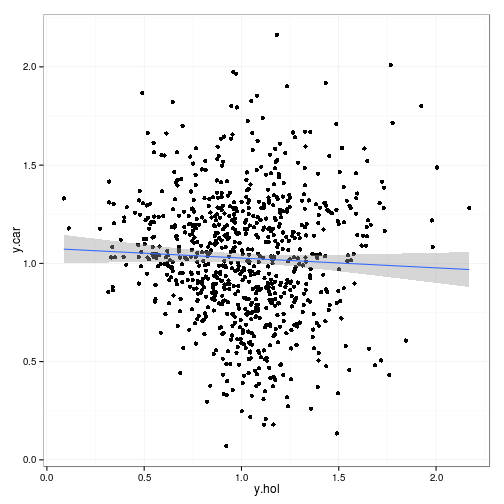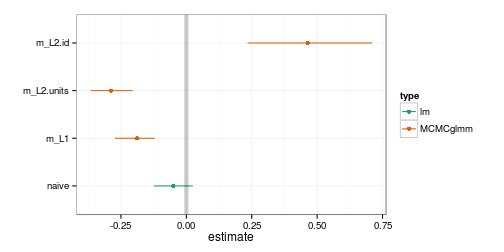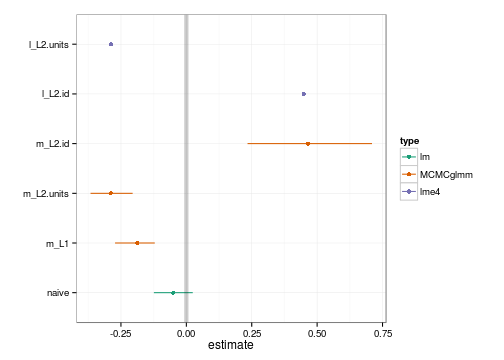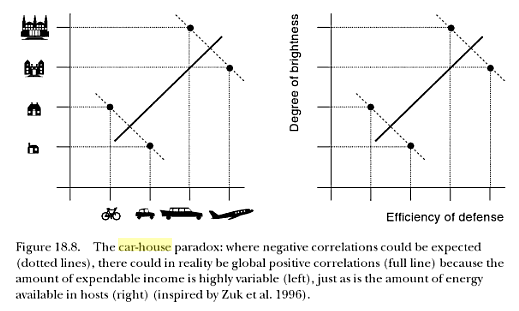# Multivariate analysis with mixed modeling tools in R

Version 2012-12-28 22:06:14

Multivariate analyses are one of the potential targets of modern mixed modeling tools. Mixed model approaches have some advantages over classical multivariate ANOVA (MANOVA) techniques; in particular, they allow for unbalanced data and relaxation of some of the usual assumptions of compound symmetry and sphericity (e.g. David Howell discusses these issues here).

In approximate order of increasing technical difficulty, one might be interested in fitting models where the different elements of the multivariate response vector are

1. exchangeable, i.e. all elements have identical variance, and the correlations between pairs of elements are all identical (this correlation structure is known as compound symmetry)
2. non-exchangeable and unstructured (i.e. no constraints on the pattern of the correlation matrix), possibly differing variances, with the distribution of individual responses chosen from the exponential family with fixed scale parameters (e.g. binomial, Poisson)
3. non-exchangeable, unstructured, normally distributed responses
4. structured normal or non-normal responses
5. multi-type responses (i.e. the elements of the response vector are drawn from different distributions, e.g. normal and Poisson)

Case #1 is easy in any modern mixed model software. It can be implemented by defining a simple intercept-level random effect associated with each vector of responses (although this approach does slightly constrain the correlation structure: the among-pair correlations must all be identical and non-negative).

(Would this list be more useful in the form of a cross-tabulation?)

Probably the most capable mixed model packages in the R ecosystem at present are nlme, lme4, and MCMCglmm.

• nlme is fairly old, but well-established and flexible. It only handles normally distributed responses (except via the glmmPQL extension in the MASS package), but it allows for a range of correlation structures for residuals (R-side effects) [cases #1, #3, #4 (normal only)]
• lme4 is newer than nlme, probably faster for large datasets, and handles GLMMs natively. R-side effects are not implemented. [cases #1, #2, #3 (with difficulty)]
• MCMCglmm is also fairly new, and handles GLMMs, multi-type models, and some R-side effects, although fewer than nlme. [cases #1, #2, #3, #4 (a few normal cases only), #5]

Here I'm going to look at case #3, especially illustrating how it can be done in MCMCglmm (easily) and lme4, with an example from the MCMCglmm “CourseNotes” vignette (vignette("CourseNotes",package="MCMCglmm")):

discuss other options … ASREML?? INLA?? ADMB??

# Preliminaries and simulating 'data'

library(MCMCglmm)
library(lme4)
library(ggplot2)
library(reshape2)
theme_set(theme_bw())


Description from the MCMCglmm course notes vignette:

Lets imagine there was only one unmeasured variable: disposable income. There are repeatable differences between individuals in their disposable income, but also some variation within individuals across the four years. Likewise, people vary in what proportion of their disposable income they are willing to spend on a holiday versus a car, but this also changes from year to year

set.seed(102)
n_indiv <- 200
n_per_indiv <- 4
n_tot <- n_indiv * n_per_indiv
id <- gl(n_indiv, n_per_indiv)  ## generate identifier columns


Per-individual wealth is normally distributed; yearly wealth is represented as an added yearly component (equal variance for each component).

av_wealth <- rlnorm(n_indiv, 0, 1)  ## avg wealth of individuals
ac_wealth <- av_wealth[id] + rlnorm(n_tot, 0, 1)  ## wealth in each year:


The fraction of wealth spent on the car varies among individuals (with a mean of 0.5); the yearly variation within individuals is distributed around this value, with a larger variance (sum of shape parameters = 2 rather than 20)

av_ratio <- rbeta(n_indiv, 10, 10)  ## 50/50, close to Gaussian
## car/holiday ratio by year (larger shape parameter/less variability)
ac_ratio <- rbeta(n_tot, 2 * av_ratio[id], 2 * (1 - av_ratio[id]))


Split wealth by ratio (not sure why we're raising to the 0.25 power, nor why y.car+y.hol doesn't add up to ac.wealth?)

y.car <- (ac_wealth * ac_ratio)^0.25
y.hol <- (ac_wealth * (1 - ac_ratio))^0.25
Spending <- data.frame(y.hol, y.car, id)

qplot(y.hol, y.car, data = Spending) + geom_smooth(method = "lm")# Models

## Naive regression

m0 <- lm(y.car ~ y.hol, data = Spending)


Results:

##      est      lwr      upr
## -0.04976 -0.12415  0.02463


Note: results can differ a fair amount depending on random-number seed (using set.seed(101) gave a much larger $$R^2$$/lower $$p$$-value)

## MCMCglmm

Replicate MCMCglmm results from the Course Notes:

m1_id <- MCMCglmm(y.car ~ y.hol, random = ~id, data = Spending, verbose = FALSE)
m1_idyr <- MCMCglmm(cbind(y.hol, y.car) ~ trait - 1, random = ~us(trait):id,
rcov = ~us(trait):units, data = Spending, family = c("gaussian", "gaussian"),
verbose = FALSE)


Compute regression coefficients for car as a function of holiday (ratios of car-holiday covariance to holiday variance at each level):

rd <- as.data.frame(m1_idyr$VCV) id.regression <- with(rd, y.car:y.hol.id/y.hol:y.hol.id) units.regression <- with(rd, y.car:y.hol.units/y.hol:y.hol.units) res_m1_id <- setNames(summary(m1_id)$solutions[2, 1:3], c("est", "lwr", "upr"))
res_m1_idyr_id <- c(est = mean(id.regression), setNames(quantile(id.regression,
c(0.025, 0.975)), c("lwr", "upr")))
res_m1_idyr_units <- c(est = mean(units.regression), setNames(quantile(units.regression,
c(0.025, 0.975)), c("lwr", "upr")))

ff <- function(x) factor(x, levels = unique(x))
combdat <- data.frame(param = ff(c("naive", "m_L1", "m_L2.units", "m_L2.id")),
type = ff(c("lm", rep("MCMCglmm", 3))), rbind(res_reg0, res_m1_id, res_m1_idyr_units,
res_m1_idyr_id))


Plot results so far:

(g1 <- ggplot(combdat, aes(param, est, ymin = lwr, ymax = upr, colour = type)) +
geom_pointrange() + labs(x = "", y = "estimate") + geom_hline(yintercept = 0,
colour = "black", lwd = 2, alpha = 0.2) + coord_flip() + scale_colour_brewer(palette = "Dark2"))(It's a little bit too bad that the data were not constructed in a way that corresponds exactly to our statistical model, so we can't be sure when we're getting the “correct” answer … ?)

## lme4

Now try to do this with lme4 – partly for the challenge, partly for the Bayes-phobic, and possibly for other advantages (e.g. speed, for very large data sets?)

The first thing we need to do is melt the data: this is done implicitly by MCMCglmm's multi-trait framework, but isn't too hard. We add a variable to index the observations in the original data set (i.e. person-years) and give the resulting derived variable the name trait for consistency with MCMCglmm

mSpending <- melt(data.frame(Spending, obs = seq(nrow(Spending))), id.var = c("obs",
"id"), variable.name = "trait")


The single-level model is easy:

L1_id <- lmer(y.car ~ y.hol + (1 | id), data = Spending)


### Approach 1 (unidentifiable)

The two-level model is not so easy. It would be tempting to try to do this as follows:

L2_unid <- lmer(value ~ trait - 1 + (0 + trait | id) + (0 + trait | obs), data = mSpending)


There's a potential problem. The two-level model that MCMCglmm is fitting is implicitly $Y_{i} \sim \text{MVN}(\mu+b_i,\Sigma_2) \\ b \sim \text{MVN}(0,\Sigma_1)$ where $$Y_{i}$$ represents the vector of observations from individual $$i$$, i.e. imposing multivariate correlation structure directly on the residuals. The individual random effects $$b_i$$ are vectors of length 2 representing the average car and holiday spending of each individual. The model fitted by lme4 is instead: $Y_{ij} \sim \text{Normal}(\mu+b_i+c_i,\sigma^2) \\ c \sim \text{MVN}(0,\Sigma_2) \\ b \sim \text{MVN}(0,\Sigma_1)$ Because lme4 doesn't natively do R-side structures, we add another grouping level – but this means that the residual variation and one of the individual-year level variances are redundant.

But it turns out that this works anyway! The unidentifiability (at least in this particular example) is not a practical problem once we process the results we get into those we want …

vc_unid <- VarCorr(L2_unid)
## transform VarCorr output into regression coefficients
vchack <- function(vc, resvar = NULL) {
vc1 <- vc$obs if (is.null(resvar)) resvar <- vc1[1, 1] diag(vc1) <- diag(vc1) + resvar res_idyr <- setNames(c(vc1["traity.hol", "traity.car"]/vc1["traity.hol", "traity.hol"], NA, NA), c("est", "lwr", "upr")) vc2 <- vc$id
res_id <- setNames(c(vc2["traity.hol", "traity.car"]/vc2["traity.hol", "traity.hol"],
NA, NA), c("est", "lwr", "upr"))
rbind(idyr = res_idyr, id = res_id)
}
vchack(vc_unid, resvar = sigma(L2_unid)^2)

##          est lwr upr
## idyr -0.2877  NA  NA
## id    0.4488  NA  NA


These are a reasonably good match with the MCMCglmm results:

##                       est     lwr     upr
## res_m1_idyr_units -0.2892 -0.3663 -0.2054
## res_m1_idyr_id     0.4649  0.2339  0.7097


## Approach 2 (fixed variance)

Alternatively, we can hack this (in development lme4) by getting the deviance function and optimizing it, but forcing one of the individual-level variances to a fixed value (it would be most convenient to fix it to zero, but then we couldn't estimate the unit-level covariance … instead we will fix it to 1.

f0 <- lmer(value ~ trait - 1 + (0 + trait | id) + (0 + trait | obs), data = mSpending)
names(getME(f0, "theta"))

##  "obs.traity.hol"            "obs.traity.car.traity.hol"
##  "obs.traity.car"            "id.traity.hol"
##  "id.traity.car.traity.hol"  "id.traity.car"

tmpf <- lmer(value ~ trait - 1 + (0 + trait | id) + (0 + trait | obs), data = mSpending,
devFunOnly = TRUE)
tmpf2 <- function(theta2) {
tmpf(c(1, theta2))
}
library(optimx)
opt1 <- optimx(par = c(0, 1, 1, 0, 1), fn = tmpf2, method = "bobyqa")


It's a little bit of a hack to reconstruct the variance-covariance matrix (could be made easier):

theta <- c(1,opt1$par$par)     ## estimated Cholesky factors
pwrss <- opt1$fvalues$fvalues  ## penalized weighted residual sum of sq
n <- nrow(mSpending)
cnms <- f0@cnms ## named list of names of variables per RE
cnms=cnms,
nc=sapply(cnms,length), ## vars per random effect term
theta=theta,
nms=names(cnms))
attr(vc,"useSc") <- TRUE
class(vc) <- "VarCorr.merMod"
vc

##  Groups   Name       Variance Std.Dev. Corr
##  obs      traity.hol 0.121    0.349
##           traity.car 0.140    0.374    -0.536
##  id       traity.hol 0.116    0.341
##           traity.car 0.152    0.389    0.393
##  Residual            0.121    0.349


Transforming as before:

lme4res <- vchack(vc)


This is a slightly bogus description (the residual variance should be added to the diagonal of the unit-level variance-covariance matrix). Furthermore, we're going to have some trouble finding the 95% confidence intervals of the regression term, which is a ratio of two things for which we will individually have trouble getting their 95% confidence intervals (we need to compute likelihood profiles). If really necessary we could reformulate the problem in terms of regression parameters, construct a wrapper for tmpf in terms of the regression parameters, and go from there.

In the meantime, though, let's try to derive the point estimates of the regression parameters from the estimates we have so far:

combdat2 <- rbind(combdat, data.frame(param = c("l_L2.units", "l_L2.id"), type = "lme4",
vchack(vc)))


Compare results:

g1 %+% combdat2## lme

library(nlme)

## Attaching package: 'nlme'

## The following object is masked from 'package:lme4':
##
## lmList

lme1 <- lme(value ~ trait - 1, random = ~trait - 1 | id, data = mSpending)


In the following model varIdent specifies different variances for each trait (response), while corSymm(form=~1|id/obs) specifies unstructured (symmetric) correlation matrices within each observation (response vector).

lme2 <- update(lme1, weights = varIdent(form = ~1 | trait), correlation = corSymm(form = ~1 |
id/obs))


The information we need is all in the model fit, but once again extracting it is a bit unwieldy:

VarCorr(lme2)

## id = pdLogChol(trait - 1)
##            Variance StdDev Corr
## traity.hol 0.02965  0.1722 trty.h
## traity.car 0.03862  0.1965 0.393
## Residual   0.06190  0.2488

vc3 <- suppressWarnings(as.numeric(VarCorr(lme2)))
## corr*stddev(hol)*stddev(car)/var(hol) covhc <-
## vc3*vc3*vc3/vc3 simpler: corr*stddev(car)/stddev(hol)
reg_id_lme <- vc3 * vc3/vc3
idyr_sdratio <- coef(lme2$modelStruct$varStruct, uncon = FALSE)
idyr_cor <- coef(lme2$modelStruct$corStruct, uncon = FALSE)
reg_idyr_lme <- idyr_sdratio * idyr_cor


lme values match the previous results:

c(id = reg_id_lme, idyr = reg_idyr_lme)

##         id idyr.y.car
##     0.4485    -0.2877


## To do

• timing comparisons? performance on simulations?